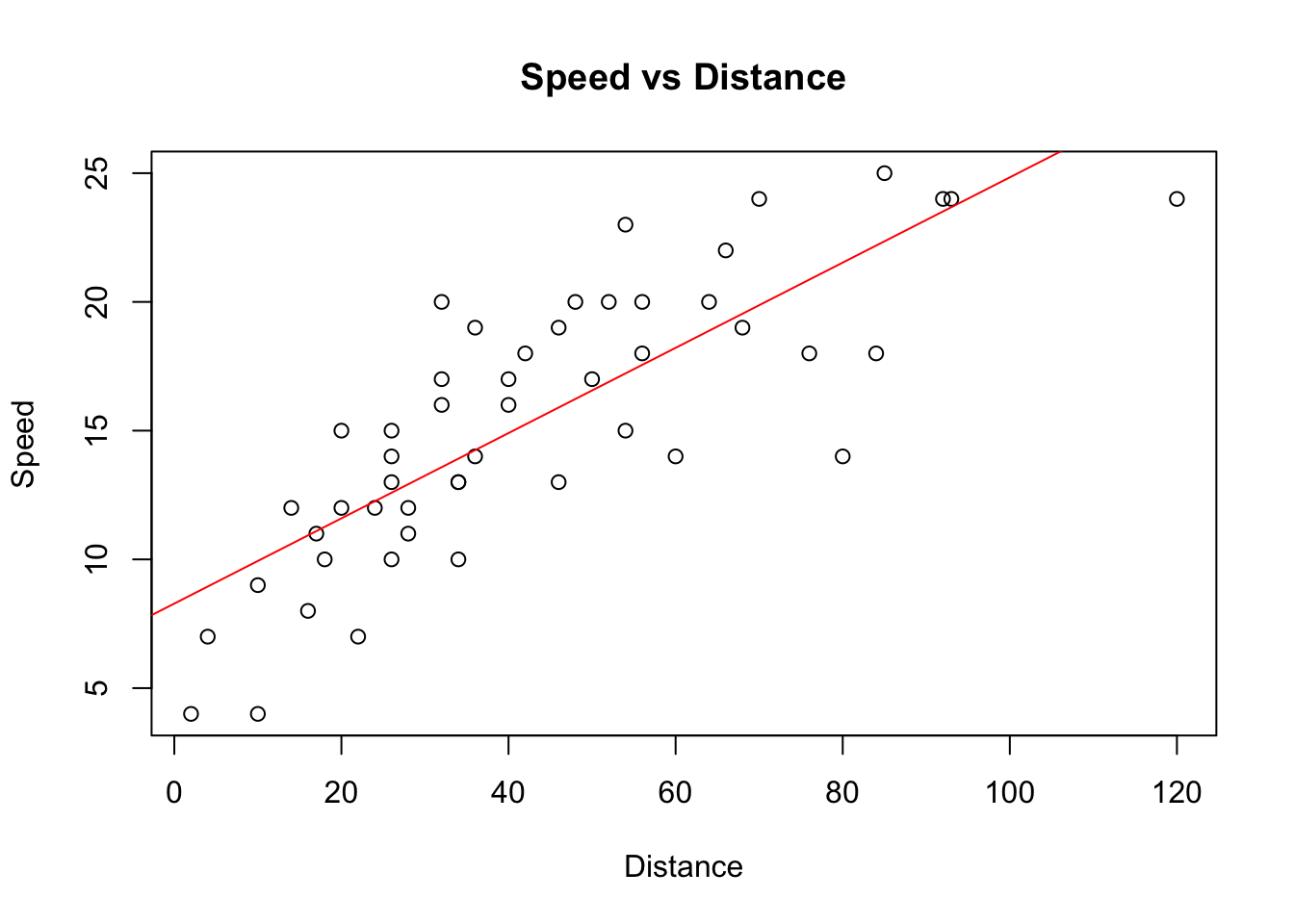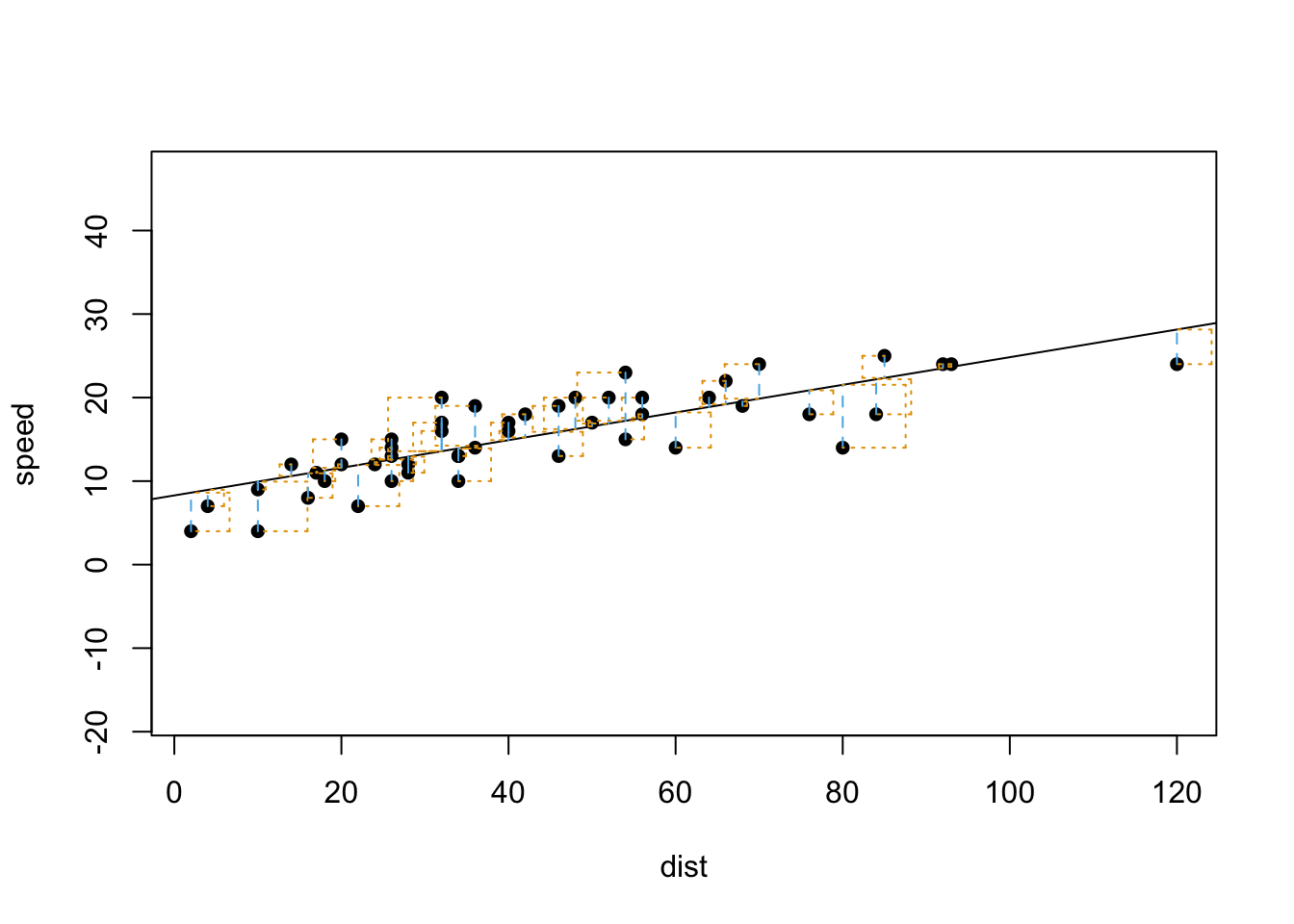### Elina Azrilyan

#### Assignment

Using the “cars” dataset in R, build a linear model for stopping distance as a function of speed and replicate the analysis of your textbook chapter 3 (visualization, quality evaluation of the model, and residual analysis.)

#### Inspecting the data

Let’s load the cars dataset and take a look at the date.

``````data(cars)
``````##   speed dist
## 1     4    2
## 2     4   10
## 3     7    4
## 4     7   22
## 5     8   16
## 6     9   10``````
``length(cars\$speed)``
``##  50``

There are 2 columns in our dataset and there are 50 rows of data.

#### Plotting the data

Scatterplot can be used to display the relationship between these 2 variables - let’s also add a regression line.

``````plot(cars\$speed ~ cars\$dist, main = "Speed vs Distance", xlab = "Distance", ylab = "Speed")
abline(lm(cars\$speed~cars\$dist), col="red") # regression line (y~x) ``````#### Identifying regression model

``````m1 <- lm(speed ~ dist, data = cars)
summary(m1)``````
``````##
## Call:
## lm(formula = speed ~ dist, data = cars)
##
## Residuals:
##     Min      1Q  Median      3Q     Max
## -7.5293 -2.1550  0.3615  2.4377  6.4179
##
## Coefficients:
##             Estimate Std. Error t value Pr(>|t|)
## (Intercept)  8.28391    0.87438   9.474 1.44e-12 ***
## dist         0.16557    0.01749   9.464 1.49e-12 ***
## ---
## Signif. codes:  0 '***' 0.001 '**' 0.01 '*' 0.05 '.' 0.1 ' ' 1
##
## Residual standard error: 3.156 on 48 degrees of freedom
## Multiple R-squared:  0.6511, Adjusted R-squared:  0.6438
## F-statistic: 89.57 on 1 and 48 DF,  p-value: 1.49e-12``````

#### Regression Model Results

Here is our regreession model:

dist = 0.166*speed + 8.284

For a good model, we typically would like to see a standard error that is at least five to ten times smaller than the corresponding coefficient. In our model the standard error is 9.5 times smaller - so there is not a lot of variability. The p-values are pretty much equal to 0 which means that both the slope and the intercept are significant. The reported R2 of 0.65 for this model means that the model explains 65 percent of the data’s variation.

#### Sum of Squares

Let’s take a look at the sum of squares.

``````suppressWarnings(suppressMessages(library(statsr)))
plot_ss(x = dist, speed, data=cars, showSquares = TRUE)````````````## Click two points to make a line.

## Call:
## lm(formula = y ~ x, data = pts)
##
## Coefficients:
## (Intercept)            x
##      8.2839       0.1656
##
## Sum of Squares:  478.021``````

#### Residuals Plot

Let’s plot residuals and see what those look like.

``plot(fitted(m1),resid(m1))``